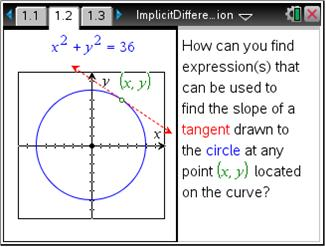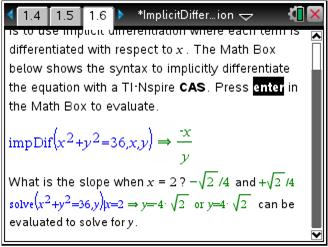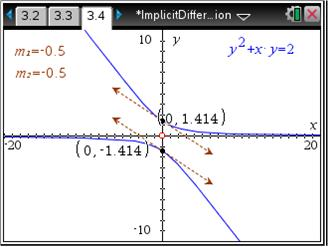# Activities

••• ##### Subject Area

• Math: Calculus: Derivatives

• ##### Author9-12

45 Minutes

• ##### Device
•TI-Nspire™ CX/CX II
•TI-Nspire™ CX CAS/CX II CAS
• TI-Nspire™
• TI-Nspire™ CAS
• ##### Software

TI-Nspire™
TI-Nspire™ CAS

3.2

• ##### Report an Issue

Implicit Differentiation

#### Activity Overview

Students find the derivative of a relation, F(x,y), that is not solved for y.

#### Key Steps

•Students will be introduced to the concept of implicit differentiation. Students will begin by solving the equation of a circle for y and then find the derivative of the relation using already familiar methods.

•Next, students will use the impDif command to find an alternate form of the derivative, and verify that the two forms are equal.

•Students will learn how to perform implicit differentiation by hand, using the impDif command to check their results. Then students will be asked to find the numerical derivative of a relation for specific x-values. A graphical connection to the results found using implicit differentiation will then be made.